Physical Chemistry : Other Electrochemistry Concepts

Example Questions

Example Question #1 : Other Electrochemistry Concepts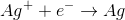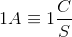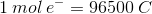A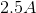current is passed through a silver ion solution. How much silver is deposited after 10 minutes?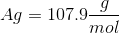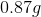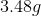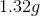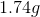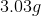Explanation:

First we need to convert minutes to seconds (10min=600s), and knowing that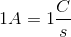, we can calculate the amount of silver deposited as follows: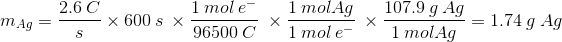If we follow the units carefully, we see that all of the units cancel out to give us a value in grams of silver, which is what we are looking for.

All Physical Chemistry Resources